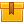# Electrical Theory for Troubleshooters Library

## Prerequisites

This curriculum is designed so that no prior knowledge is required.

## Description

This library consists of twelve that are excellent for the training of electricians and electronic technicians, as well as for the multi-craft training needs of process and manufacturing facilities.

## Objectives

Learn the basics of electricity, circuits, magnetism, inductance, capacitance 3-phase circuits, wye and delta connections, and transformers.

## ContentThis is the first lesson in the iKNOW™ Electrical Theory for
Troubleshooters Library. This lesson uses animation to demonstrate
atomic structure, electricity, and how a simple circuit operates. The
lesson also explains the characteristics of good conductors and
insulators.

•Introduction to ElectricityThis is the second lesson in the iKNOW™ Electrical Theory for
Troubleshooters Library. This lesson covers Ohm’s Law and the use of
Ohm’s Law to calculate an unknown value. It also defines voltage,
current, resistance, and power.

•Basic Electrical PropertiesThis is the third lesson in the iKNOW™ Electrical Theory for
Troubleshooters Library. The lesson presents the operation of a series
circuit and trains participants in the identification of simple
schematic symbols used to represent components in a series circuit. The
behavior of current, resistance, and current in a series circuit, and
the used of Kirchhoff’s Voltage Law to find total voltage are also
covered.

•Series CircuitsThis is the fourth lesson in the iKNOW™ Electrical Theory for
Troubleshooters Library. This lesson describes the behavior of voltage,
current, and resistance in a parallel circuit. The learner is also
instructed in the identification of the series and parallel portions of a
series-parallel circuit.

•Parallel CircuitsThis is the fifth lesson in the iKNOW™ Electrical Theory for
Troubleshooters Library. This lesson teaches the basic AC
characteristics of voltage, including how voltage changes over time. The
participant is also instructed in using sine waves to interpret the
frequency of

AC voltage.

•Alternating CurrentThis sixth lesson in the iKNOW™ Electrical Theory for Troubleshooters
Library uses animations and demonstrations to explain the principles of
magnetism, including flux density and electromagnetic induction. The
lesson also shows how to plot a sine wave using a graph.

•ElectromagnetismThis is the seventh lesson in the iKNOW™ Electrical Theory for
Troubleshooters Library. This lesson builds on the information presented
in the lesson, Electromagnetism. Types of induction, phase, and the
effect of induction in AC circuits are covered.

•InductanceThis is the eighth lesson in the iKNOW™ Electrical Theory for
Troubleshooters Library. This lesson explains capacitors, their
function, and how capacitance affects AC circuits.

•CapacitanceThis is the ninth lesson in the iKNOW™ Electrical Theory for
Troubleshooters Library. This lesson defines 3-phase AC, describes the
components and operating principle of 3-phase generators, and using the
formula for frequency, shows how rotor speed and the number of poles is
related to frequency.

•Three-Phase CircuitsThe tenth lesson in the iKNOW™ Electrical Theory for Troubleshooters
Library, this lesson discusses Wye and Delta configurations and explains
the relationship between phase and line voltages in various
connections, and demonstrates the application of the formula that shows
this relationship.

•Wye Delta ConnectionsThe eleventh lesson in the iKNOW™ Electrical Theory for Troubleshooters
Library, this lesson presents the basic parts of a transformer and their
function. The lesson explains turns ratio and its relationship to a
transformer’s input and output voltages. The participant is also
cautioned regarding the dangers of improper transformer connections.

•Introduction to TransformersThis is the final lesson in the iKNOW™ Electrical Theory for
Troubleshooters Library. This lesson builds on the information presented
in the lesson, Introduction to Transformers.  How to determine primary current and voltage, secondary current and
voltage, and load is taught. The function of various transformers is
also explained.

•ETFT Transfomers

0
0 Reviews